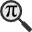## General

Display information for equation id:math.286781.10 on revision:286781

* Page found: Talk:Lagrangian (eq math.286781.10)

(force rerendering)

Cannot find the equation data in the database. Fetching from revision text.

Occurrences on the following pages:

TeX (original user input):

\delta


TeX (checked):

\delta


### LaTeXML (experimental; uses MathML) rendering

MathML (482 B / 255 B) :${\displaystyle\delta}$
<math xmlns="http://www.w3.org/1998/Math/MathML" id="p1.1.m1.1" class="ltx_Math" alttext="{\displaystyle\delta}" display="inline">
<semantics id="p1.1.m1.1a">
<mi id="p1.1.m1.1.1" xref="p1.1.m1.1.1.cmml">δ</mi>
<annotation-xml encoding="MathML-Content" id="p1.1.m1.1b">
<ci id="p1.1.m1.1.1.cmml" xref="p1.1.m1.1.1">𝛿</ci>
</annotation-xml>
<annotation encoding="application/x-tex" id="p1.1.m1.1c">{\displaystyle\delta}</annotation>
</semantics>
[/itex]


SVG (1.075 KB / 644 B) :

### MathML with SVG or PNG fallback (recommended for modern browsers and accessibility tools) rendering

SVG image empty. Force Re-Rendering

SVG (0 B / 8 B) :

PNG (0 B / 8 B) :

$\delta$## Translations to Computer Algebra Systems

### Translation to Maple

In Maple: delta

\delta: Could be the first Feigenbaum constant.

But this system don't know how to translate it as a constant. It was translated as a general letter.


### Translation to Mathematica

In Mathematica: \[Delta]

\delta: Could be the first Feigenbaum constant.

But this system don't know how to translate it as a constant. It was translated as a general letter.


## Similar pages

Calculated based on the variables occurring on the entire Talk:Lagrangian page

## Identifiers

• $\delta$### MathML observations

0results

0results

no statistics present please run the maintenance script ExtractFeatures.php

0 results

0 results